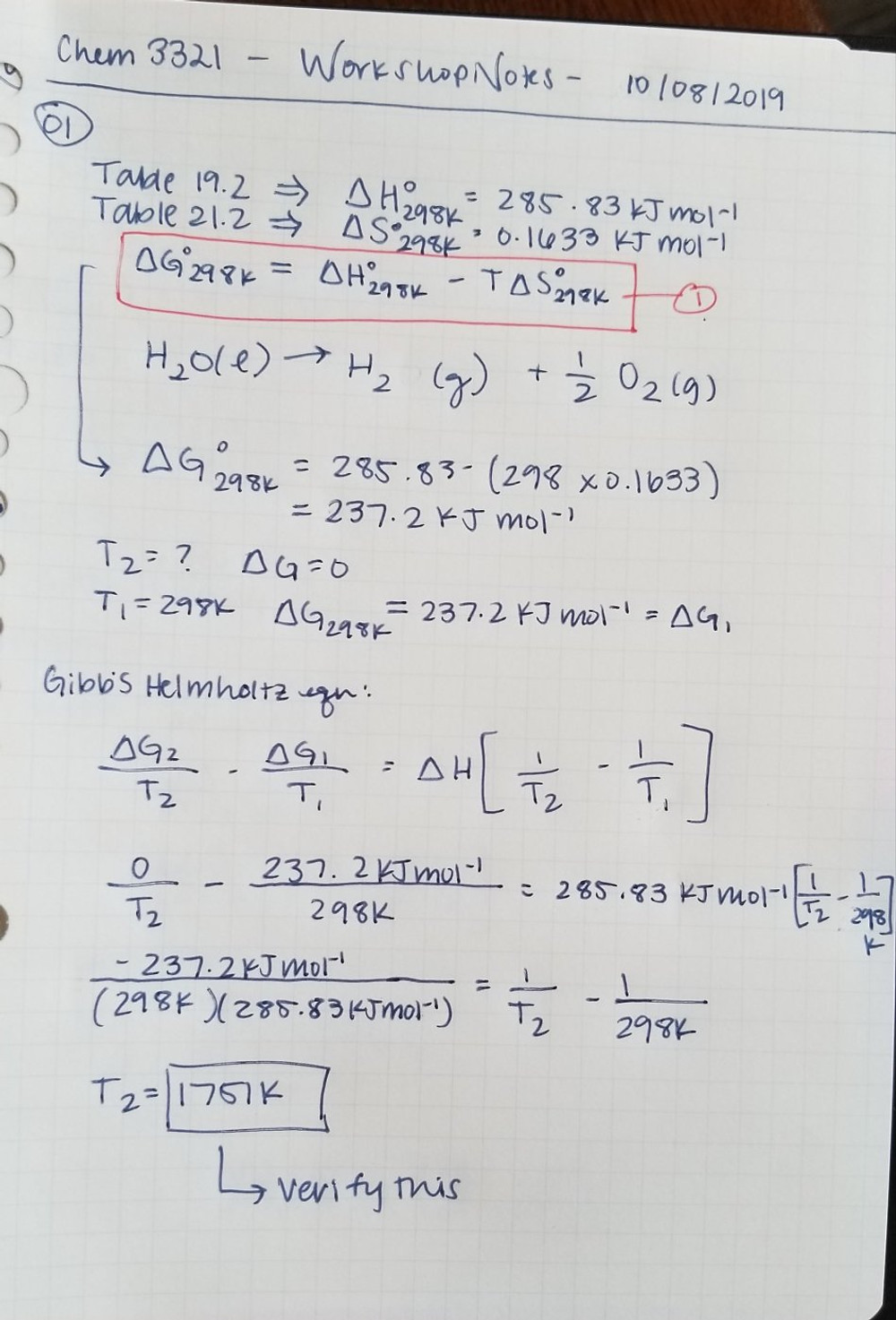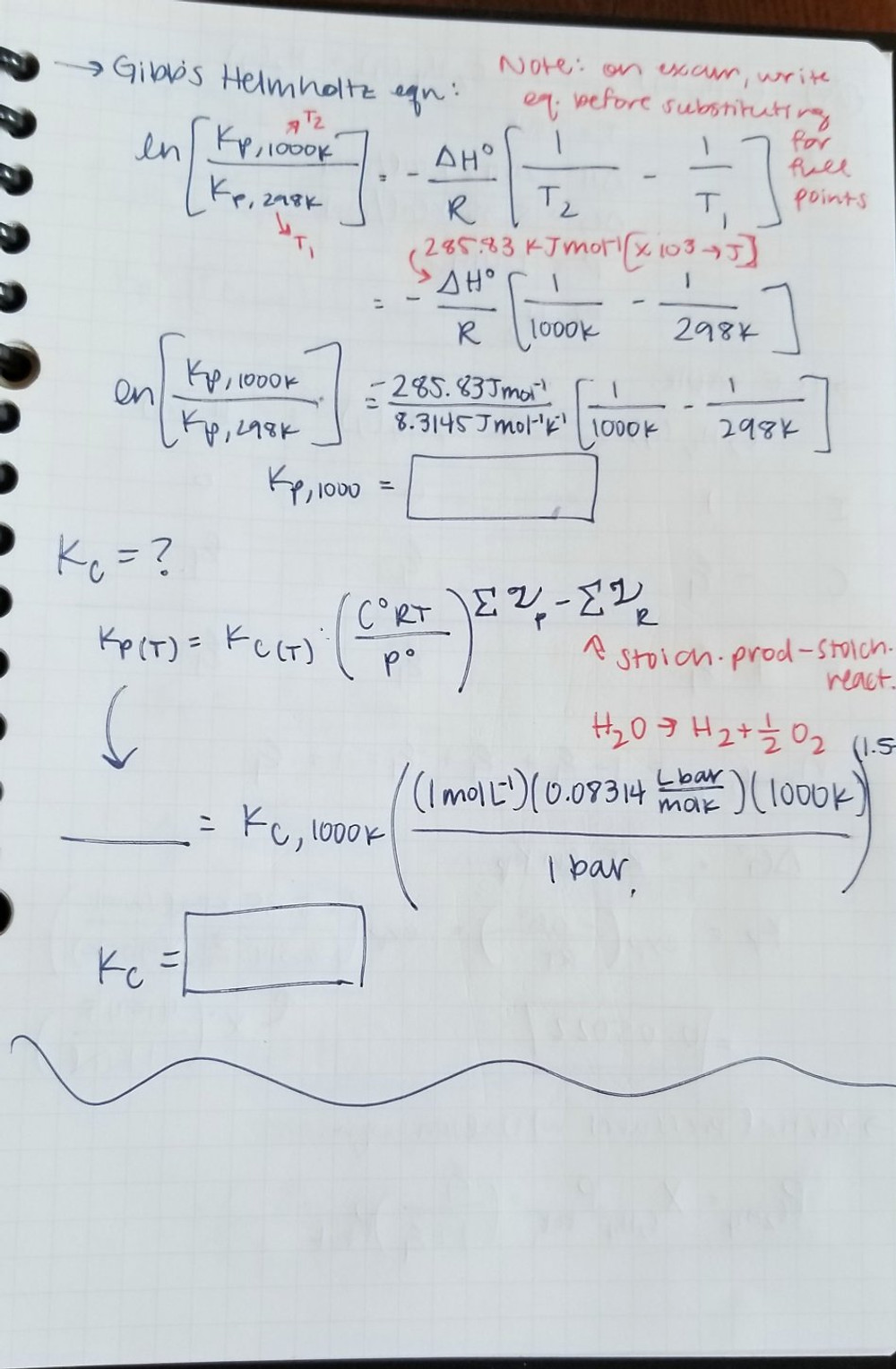# PCHEM Workshop 6 Notes

Updated: Dec 30, 2019

Sixth workshop with Dineli for Physical Chemistry (CHEM 3321) with Dr. Nielsen at UT Dallas!

### WORKSHOP WITH DINELI | October 8, 2019

Problem 1 – chemical reaction

Using tabulated ∆fH◦ 298 (Table 19.2) and standard molar entropies given in Table 21.2, calculate ∆rxnG◦ 298 for the reaction H2O(`)→H2(g) + 1 2O2(g) At what temperature would the reaction become favorable assuming ∆rxnH◦ 298 is independent of temperature?

Problem 2 – derivative manipulations

Starting from Equation 22.34, show thatProblem 3

(a) Using Table 26.1 find KP for the following reaction at 298 K and 1 bar N2O4(g) → 2NO2(g) (b) Solve for ξ, the extent of reaction, assuming one mole of reactant and no product is initially present.

Problem 4

Using the data in Table 19.2 find KP and KC for the reaction in problem 1 at 1000 K and 1 bar. Rationalize your answer in terms of Le Chatelier’s Principle.

Problem 5

At 900 K, the reaction C2H6(g) → C2H4(g) + H2(g) has ∆H◦ = 24.42 kcal/mol and ∆G◦ = 5.35 kcal/mol. Calculate the percent H2 present at equilibrium if pure C2H6 is passed over a dehydrogenation catalyst at this temperature and 1 atm pressure. (b) Estimate the percent H2 at equilibrium at 1000 K.

Problem 6

Suppose that we have a mixture of the gases H2(g), CO2(g), CO(g) and H2O(g) at 1260 K, with P(H2) = 0.3 bar, P(CO2) = 0.5 bar, P(CO) = 2.5 bar and P(H2O) = 0.5 bar. Is the reaction described by the equation H2(g) + CO2(g) → CO(g) + H2O(g) KP = 1.59 at equilibrium under these conditions? If not what direction will the reaction proceed to attain equilibrium?